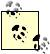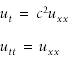# Introduction

Partial differential equations are incredibly important in scientific and engineering problem solving, and it's no wonder a vast array of methods have been devised to solve them. As in the case of ordinary differential equations, you can divide problems involving partial differential equations into two broad classes: boundary value problems and initial value problems (including initial-boundary value problems).

The best examples for boundary value problems are elliptic equations of the form:I'm using the standard subscript notation here to indicate partial derivatives, e.g., ux represents the first partial derivative of u with respect to x.

The first equation shown above is the well-known Laplace equation, while the second is the Poisson equation. Boundary conditions are required for these equations and can consist of specification of u, derivatives of u, or a mix of these two on the problem boundary, corresponding to Dirichlet, Neumann, and mixed conditions, respectively. The numerical solution to boundary value problems of this form generally consists of discretizing the problem domain using one of a variety of techniques, such as the finite difference method, finite element method, or boundary element method, and formulating a system of algebraic equations that can be solved for the unknown values of u at discrete nodal points inside the problem domain or boundary.

Time does not enter into elliptic problems, so the solution sought is a steady state or static solution. For example, a fluid flow problem can be formulated as a steady state problem, or a structural problem can be formulated as a static problem. The resulting system of equations may or may not be linear and, depending on the size of the problem and how it's discretized, could be a relatively small system of equations or a very large one. These factors combine to guide selection of an appropriate solution algorithm. Small linear systems can be solved directly, but nonlinear or large systems might require an iterative approach.

Time-dependent problems are typically of the form:The first equation is the one-dimensional diffusion equation, and the second is the one-dimensional wave equation. These equations can, of course, apply to higher-dimensional problems as well, with the addition of suitable terms. In terms of standard mathematical classifications, the diffusion equation represents a parabolic equation, while the wave equation represents a hyperbolic equation.

An important distinction between these equations and the previously mentioned elliptic equations is the dependence on time in the case of parabolic and hyperbolic equations . This has implications with regard to the method of solution. In time-dependent problems, marching or stepping algorithms can be used to propagate solutions in time, just as you saw Chapter 11 for time-dependent ordinary differential equations. Further, in addition to boundary conditions of the types mentioned earlier, you also have to specify initial conditions for time-dependent problems.

Partial differential equations apply to an incredible variety of problems, from describing physical processes to modeling economic processes, and all sorts of problems in between. For many problems, exact solutions are difficult if not impossible to find, and numerical methods play an important role in their solution. Given the ready availability of computing power these days, numerical methods for partial differential equations are enjoying much attention and rapid development. It seems the number and variety of methods is almost as varied as are the problems being solved.

In general, Excel will do nothing to formulate a solution to any given partial differential equation. You have to do the math yourself. However, once you have formulated a solution in the form of a nonlinear equation or system of linear or nonlinear equations, either static or time-dependent, you can leverage Excel to help solve these equations. In this regard, the recipes in this chapter provide several examples of how you might use Excel to solve partial differential equations. These are by no means all-inclusive, but I hope they provide some inspiration for taking advantage of Excel's features to help solve some complicated problems.Excel Scientific and Engineering Cookbook (Cookbooks (OReilly))
ISBN: 0596008791
EAN: 2147483647
Year: N/A
Pages: 206
Authors: David M Bourg